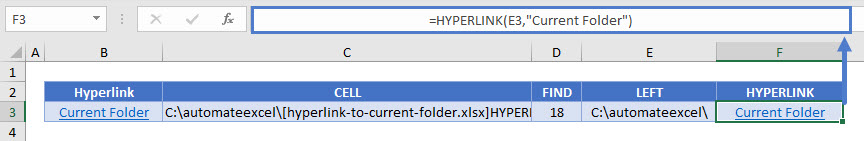# Hyperlink to Current Folder in Excel

This tutorial will demonstrate how to create a hyperlink to the current folder in Excel.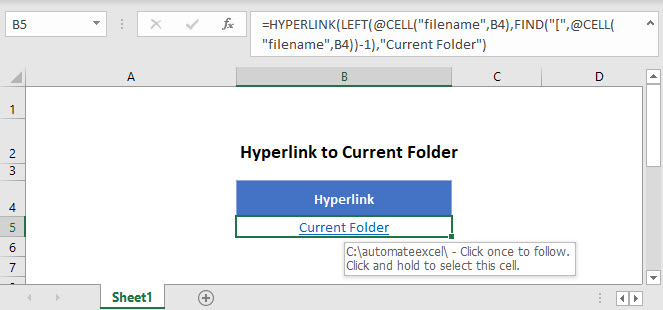## Create Hyperlink to Current Folder

With the HYPERLINK function you can easily create a hyperlink to a folder. Its syntax is: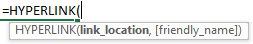To hyperlink to the current folder we will use the following formula:

``=HYPERLINK(LEFT(CELL("filename",B2),FIND("[",CELL("filename",B2))-1),"Current Folder")``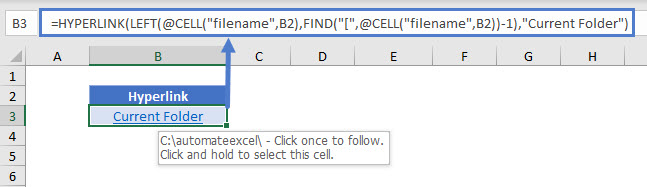Let’s see how this formula works.

### CELL Function

We can use the CELL Function to return the file path, name, and sheet by inputting “filename”.

``=CELL(“filename”,B2)``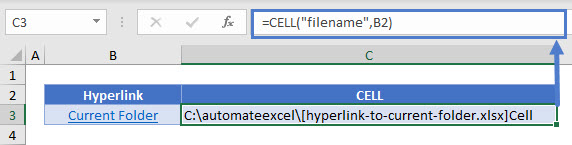This function will always return the sheet name also together with the path and the file name in brackets. Let’s see how to remove the unnecessary parts to get only the path name.

### FIND Function

First we determine the position of the opening bracket “[” before the file name with the FIND function.

``=FIND("[",CELL("filename",B2))``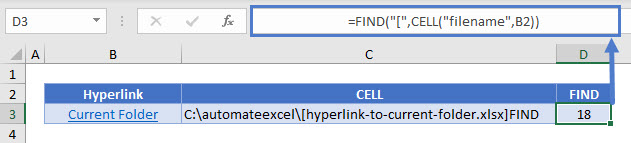### LEFT Function

Once we have the position of the opening bracket “[” before the file name (i.e. the starting position of the unnecessary part), we can use it for passing it to the LEFT function to extract only the path name.

``=LEFT(CELL("filename",B2),FIND("[",CELL("filename",B2))-1)``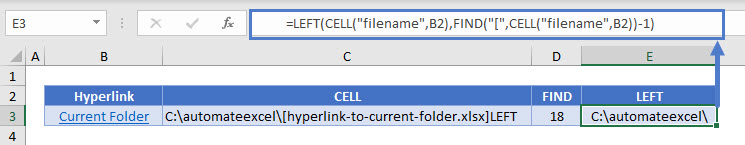``=HYPERLINK(E3,"Current Folder")``